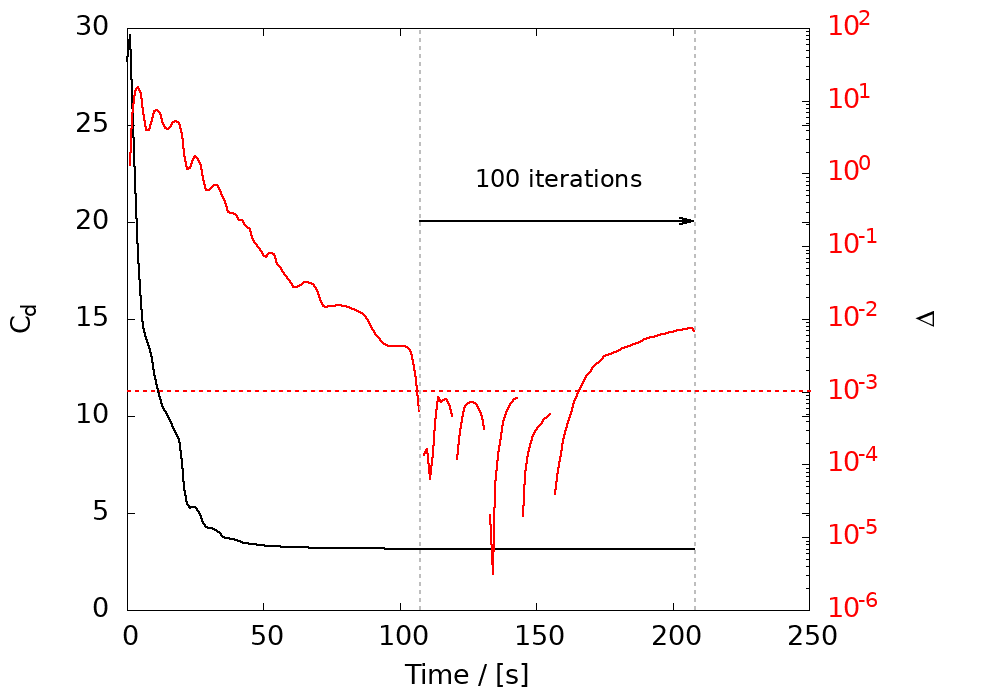The runTimeControl function object provides a mechanism to manipulate cases based on a set of run-time conditions. On satisfying the condition(s) users can instruct the case to:

• terminate and write calculation data to disk, or
• start additional function objects by setting triggers

The set of run-time conditions comprise:

• average: average value converges to within limits;
• equationInitialResidual: equation initial residual exceeds a value;
• equationMaxIter: equation number of iterations exceeds a value;
• maxDuration: maximum duration;
• minMax: minimum/maximum exceeds limits;
• minTimeStep: minimum time step exceeds a value;
• none: no-op.

By default, cases will terminate if any condition is satisfied using an OR condition. Optionally, conditions can be grouped whereby the case will only be terminated if all group member conditions are satisfied using an AND condition.

The optional nWriteStep entry can be used to write all field data each time the conditions are satisfied for a specified number of steps prior to termination.

## Usage🔗

The function object is specified using:

runTimeControl1
{
type            runTimeControl;
libs            ("libutilityFunctionObjects.so");
conditions
{
<list of conditions>
}
}


The following example will terminate the calculation if:

• the momentum initial residual exceeds 0.9 (used as a divergence indicator), OR
• the number of the momentum solver iterations exceeds 100 (used as a divergence indicator), OR
• if the average drag coefficient remains within a 0.001 range over a window of 20 steps AND the maximum momentum equation residual falls below 1e-4.

The grouping is achieved using the groupID entry where conditions with the same group value will be combined using an AND operation. Any integer value can be used.

runTimeControl1
{
type            runTimeControl;
libs            (utilityFunctionObjects);

conditions
{
condition0
{
type            equationInitialResidual;
fields          (U);
value           0.7;
mode            maximum;
}
condition1
{
type            equationMaxIterCondition;
fields          (U);
threshold       100;
}
condition2
{
type            average;
functionObject  forceCoeffs1;
fields          (Cd);
tolerance       1e-3;
window          20;

groupID         1;          // Note grouping
}
condition3
{
type            equationInitialResidual;
fields          (U);
value           1e-04;
mode            minimum;

groupID         1;          // Note grouping
}
}
}


The next example is based on an external aerodynamics case where:

• Using runTimeControl1, after the average drag coefficient settles to within a range of 1e-3 based on an exact window of size 20, trigger 1 is set.
• Trigger 1 activates the fieldAverage1 and runTimeControl2, where the latter uses a maxDuration condition to terminate the run after a further 100 steps.
fieldAverage1
{
type            fieldAverage;
libs            (fieldFunctionObjects);
triggerStart    1;
timeStart       500;
controlMode     timeOrTrigger;
writeControl    writeTime;
fields
(
U
{
base        iteration;
mean        on;
prime2Mean  off;
}
);
}

runTimeControl1
{
type            runTimeControl;
libs            (utilityFunctionObjects);
conditions
{
condition1
{
type            average;
functionObject  forceCoeffs1;
fields          (Cd);
tolerance       1e-3;
window          20;
windowType      exact;
}
}
satisfiedAction setTrigger;
trigger         1;
}

runTimeControl2
{
type            runTimeControl;
libs            (utilityFunctionObjects);

controlMode     trigger;
triggerStart    1;
conditions
{
condition1
{
type            maxDuration;
duration        100;
}
}
satisfiedAction end;
}


## Sample output🔗

The following image shows a time history of the drag coefficient and change in drag coefficient:At time=108 the runTimeControl1 average condition is satisfied when the change falls below 0.001. This activates runTimeControl2 that instructs the code to continue for a further 100 iterations, after which the calculation terminates.

## Further information🔗

Source code:

API:

Tutorial

History:

• Introduced in version v3.0+